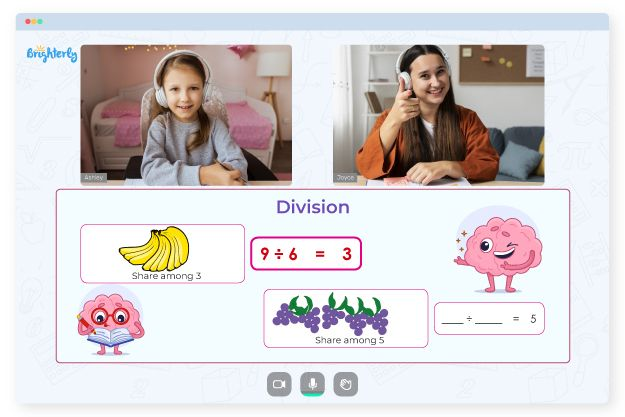# Equivalent Fractions Worksheets

Students in the second grade and beyond start dealing with fractions and learn to identify, compare, and tell their equivalents. Worksheets help children understand advanced fraction problems like equivalent fractions. Exercises in worksheets can be multiple choice questions or equations depending on the students’ familiarity with the topic. Equivalent fractions worksheet contains questions that are challenging enough for students to solve and not too tricky so that kids do not abandon them.

## Benefits of Using Equivalent Fraction Worksheet

Equivalent fractions worksheets are beneficial for children and allow them to learn the topic in a simplified format. Parents may give their children access to free and printable worksheets to foster the learning process.### Choose the Equivalent Fraction

Equivalent Fractions Worksheet### Make Equivalent Fractions

Equivalent Fraction Worksheet### Patterns of Equivalent Fractions

Free Equivalent Fractions Worksheets### Cost-effectiveness

Free equivalent fractions worksheets are easily accessible resources that are helpful for children. Kids can use them at home, school, or wherever. All parents or teachers need is to print several copies of free PDF versions, which won’t cost more than a couple of dollars.

### Learner-friendliness

Equivalent fractions worksheets contain visual simulations that help children picture what they learn. These visuals are learner-friendly and make children feel at ease as they study. Such exercises encourage evaluative thinking and increase a child’s interest in learning the topic.

Math for Kids

Is Your Child Struggling With Math?
1:1 Online Math Tutoring### Children’s Ability to Learn at Their Pace

Worksheets provide a safe environment for kids to learn more efficiently and without pressure. A standard equivalent fractions worksheet encourages students to learn fractions steadily. It doesn’t breed destructive competition in the classroom, which can hinder kids from learning the ins and outs of equivalent fractions in a relaxed atmosphere.

### Promotion of Interactive Learning

Equivalent fractions worksheets follow a stepwise learning method. With the help of tutors and peers, students understand the characters in the worksheets, which is the first step to correctly identify the equivalent forms of fractions. Worksheets also contain interactive activities, such as games and puzzles, to keep children interested in learning the subject.

### Encouragement of Repetitive Learning

Brighterly’s equivalent fractions worksheets encourage repetitive learning. The system helps a child retain what they have learned. Constant practice with worksheets increases a child’s chances of perfecting knowledge of equivalent fractions and being confident enough to face any question on the topic.

An excellent free equivalent fractions worksheet will help children learn as long as they practice consistently. It consists of various question formats that are kid-friendly. And in case you decide to use equivalent fractions worksheets in PDF, you can print as many copies as you need for practice and lessons.

### Fractions Worksheets

Problems with Division?• Try lessons with an online tutor.

Is your child having difficulties mastering the basics of division? An online tutor could be the solution.